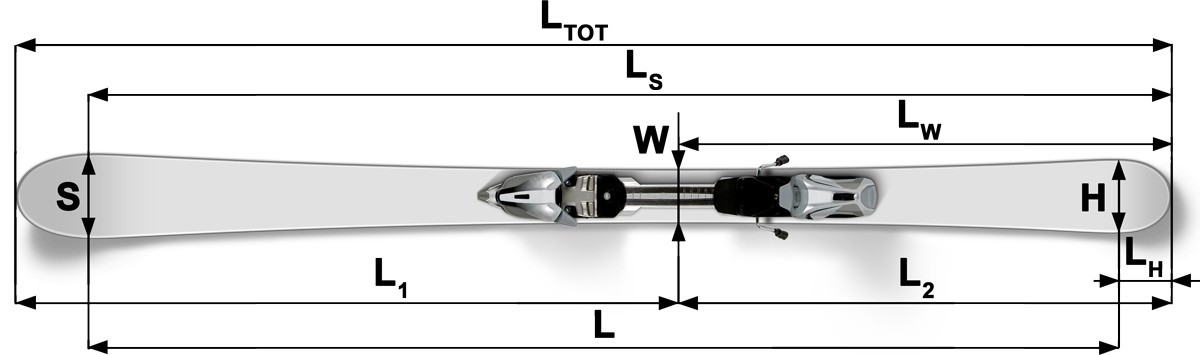### Determination of ski length and side cut radius

#### Ski length

To control the ski length, the developed length (flat material length) is used.

The rear and front ski lengths are measured starting from the narrowest point of the ski. In order to avoid that the entire side cut radius becomes distorted by the different geometries of the tail and tip, the rear ski width is recorded at 90% of the measured rear ski length whilst the front ski width is recorded at 80% of the measured front ski length.

The length is measured using a measuring tape with a precision of 1 mm. To avoid rounding differences the width can be measured by using calipers providing a precision of 1/100 mm. Width values S, H and W will be rounded in accordance with standard commercial practice to 1/10 mm and R is calculated with rounded values.LTOT, LW, LS, L1, L2, S, H and W must be or are given in millimeter (mm).

The side cut radius R is calculated by using the following formula:

LH = LW * 0.1

L1 = (LTOT - LW) * 0.8

L2 = LW * 0.9

LS = L1 + LW

L = L1 + L2

 R = L2 2000 * (S + H - 2 * W)

R is given in m.

If the side cut radius lies below the valid limit set by the rules after the first measurement, the measurement including the calculations must be repeated three times. The arithmetic mean XR calculated from these three side cut measurements. To account for measurement errors in the length and width, the side cut radius is recorded as 1.015 XR. This figure will reflect a measurement error of 1.5% on average. 1.015 XR must be greater than or equal to the valid radius limit set by the rules.

Since the formula of the existing measuring method intends to reflect the unadulterated (undistorted) measurement of a side cut radius, this assumes that a continuously differentiable and monotonously curvedradius shall be maintained between point "S" and point "H", i.e. without any turning point in-between those two points.

In order to reflect the above, it is clarified thatthe radius determination assumes that:

At any point on the distance from W to H the width has to be smaller than at H.

At any point on the distance from W to S the width has to be smaller than at S.

If any of the above assumptions are not fulfilled, the concerned ski cannot be found as conform to the specifications.

### Ski Calculator as offline web app for mobile devices

You also can use Ski Calculator as an offline web app for mobile devices.

1. Add this web app as bookmark to your home screen. You will get an icon on your home screen.
2. Start the web app once if you are connected to internet to load all data for offline use.
3. Now you can also use the web app offline.

FIS Ski Radius Calculator version 2.0

© FIS International Ski Federation - 2013